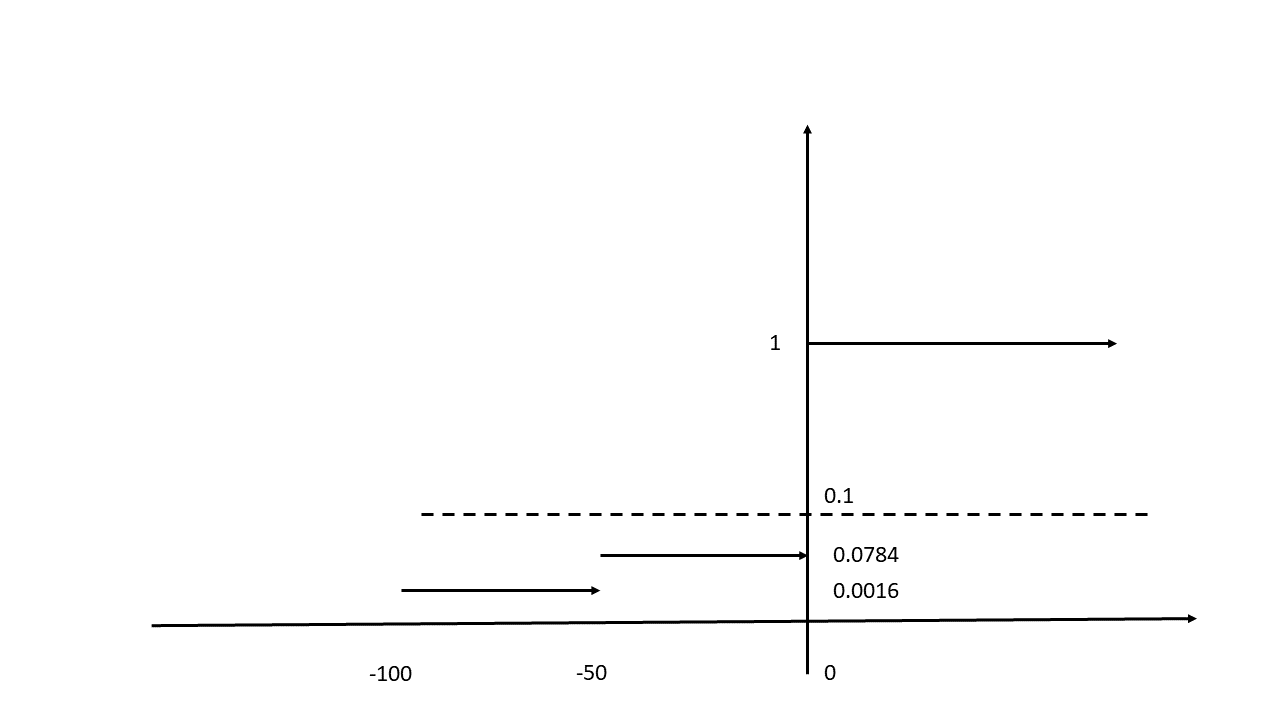24
Jun 20

## Solution to Question 2 from UoL exam 2018, Zone B

There are three companies, called A, B, and C, and each has a 4% chance of going bankrupt. The event that one of the three companies will go bankrupt is independent of the event that any other company will go bankrupt.

Company A has outstanding bonds, and a bond will have a net return of$r = 0\%$ if the corporation does not go bankrupt, but it will have a net return of$r = -100\%$, i.e., losing everything invested, if it goes bankrupt. Suppose an investor buys $1000 worth of bonds of company A, which we will refer to as portfolio${P_1}$. Suppose also that there exists a security whose payout depends on the bankruptcy of companies B and C in a joint fashion. In particular, if neither B nor C go bankrupt, this derivative will have a net return of$r = 0\%$. If exactly one of B or C go bankrupt, it will have a net return of$r = -50\%$, i.e., losing half of the investment. If both B and C go bankrupt, it will have a net return of$r = -100\%$, i.e., losing the whole investment. Suppose an investor buys$1000 worth of this derivative, which is then called portfolio${P_2}$.

(a) Calculate the VaR at the$\alpha = 10\%$ critical level for portfolios$P_1$ and${P_2}$. [30 marks]

Independence of events. Denote$A,{A^c}$ the events that company A goes bankrupt and does not go bankrupt, resp. A similar notation will be used for the other two companies. The simple definition of independence of bankruptcy events$P(A \cap B) = P(A)P(B)$ would be too difficult to apply to prove independence of all events that we need. A general definition of independence of variables is that their sigma-fields are independent (it will not be explained here). This general definition implies that in all cases below we can use multiplicativity of probability such as$P(B \cap C) = P(B)P(C) = {0.04^2} = 0.0016,\,\,P({B^c} \cap {C^c}) = {0.96^2} = 0.9216,$$P((B \cap {C^c}) \cup ({B^c} \cap C)) = P(B \cap {C^c}) + P({B^c} \cap C) = 2 \times 0.04 \times 0.96 = 0.0768.$

The events here have a simple interpretation: the first is that “both B and C fail”, the second is “both B and C fail”, and the third is that “either (B fails and C does not) or (B does not fail and C does)” (they do not intersect and additivity of probability applies).

Let${r_A},{r_S}$ be returns on A and the security S, resp. From the problem statement it follows that these returns are described by the tables
Table 1${r_A}$${r_A}$ Prob 0 0.96 -100 0.04

Table 2${r_S}$${r_S}$ Prob 0 0.9216 -50 0.0768 -100 0.0016

Everywhere we will be working with percentages, so the dollar values don’t matter.

From Table 1 we conclude that the distribution function of return on A looks as follows:Figure 1. Distribution function of portfolio A

At$x=-100$ the function jumps up by 0.04, at$x=0$ by another 0.96. The dashed line at$y=0.1$ is used in the definition of the VaR using the generalized inverse:$VaR_A^{0.1} = \inf \{ {x:{F_A}(x) \ge 0.1}\} = 0.$

From Table 2 we see that the distribution function of return on S looks like this:The first jump is at$x=-100$, the second at$x=-50$ and third one at$x=0$. As above, it follows that$VaR_S^{0.1} = \inf\{ {x:{F_S}(x) \ge 0.1}\} = 0.$

(b) Calculate the VaR at the$\alpha=10\%$ critical level for the joint portfolio${P_1} + {P_2}$. [20 marks]

To find the return distribution for$P_1 + P_2$, we have to consider all pairs of events from Tables 1 and 2 using independence.

1.$P({r_A}=0,{r_S}=0)=0.96\times 0.9216=0.884736$

2.$P({r_A}=-100,{r_S}=0)=0.04\times 0.9216=0.036864$

3.$P({r_A}=0,{r_S}=-50)=0.96\times 0.0768=0.073728$

4.$P({r_A}=-100,{r_S}=-50)=0.04\times 0.0768=0.003072$

5.$P({r_A}=0,{r_S}=-100)=0.96\times 0.0016=0.001536$

6.$P({r_A}=-100,{r_S}=-100)=0.04\times 0.0016=0.000064$

Since we deal with a joint portfolio, percentages for separate portfolios should be translated into ones for the whole portfolio. For example, the loss of 100% on one portfolio and 0% on the other means 50% on the joint portfolio (investments are equal). There are two such losses, in lines 2 and 5, so the probabilities should be added. Thus, we obtain the table for the return$r$ on the joint portfolio:

Table 3$r$$r$ Prob 0 0.884736 -25 0.073728 -50 0.0384 -75 0.003072 -100 0.000064

Here only the first probability exceeds 0.1, so the definition of the generalized inverse gives$VaR_r^{0.1} = \inf \{ {x:{F_r}(x) \ge 0.1}\} = 0.$

(c) Is VaR sub-additive in this example? Explain why the absence of sub-additivity may be a concern for risk managers. [20 marks]

To check sub-additivity, we need to pass to positive numbers, as explained in other posts. Zeros remain zeros, the inequality$0 \le 0 + 0$ is true, so sub-additivity holds in this example. Lack of sub-additivity is an undesirable property for risk managers, because for them keeping the VaR at low levels for portfolio parts doesn’t mean having low VaR for the whole portfolio.

(d) The expected shortfall$E{S^\alpha }$ at the$\alpha$ critical level can be defined as$ES^\alpha= - E_t[R|R < - VaR_{t + 1}^\alpha],$

where$R$ is a return or dollar amount. Calculate the expected shortfall at the$\alpha = 10\%$ critical level for portfolio$P_2$. Is this risk measure sub-additive? [30 marks]

Using the definition of conditional expectation and Table 3, we have (the time subscript can be omitted because the problem is static)$ES^{0.1}=-E[r|r$=-\frac{-25\times 0.073728-50\times 0.0384-75\times 0.003072-100\times 0.000064}{0.073728+0.0384+0.003072+0.000064}=\frac{4}{0.115264}=34.7029.$

There is a theoretical property that the expected shortfall is sub-additive.